• +91 9971497814
• info@interviewmaterial.com

# RD Chapter 33- Probability Ex-33.4 Interview Questions Answers

### Related Subjects

Question 1 :
If A and B be mutually exclusive events associated with a random experiment such that P (A) = 0.4 and P (B) = 0.5, then find:
(i) P(A ∪ B)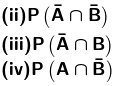Given: A and B are twomutually exclusive events.

P (A) = 0.4 and P (B)= 0.5

By definition ofmutually exclusive events we know that:

P (A  B) = P (A) + P (B)

Now, we have to find

(i) P (A  B) = P (A) + P (B) = 0.5 + 0.4 = 0.9

(ii) P (A′ ∩ B′)= P (A  B)′ {usingDe Morgan’s Law}

P (A′ ∩ B′)= 1 – P (A  B)

= 1 – 0.9

= 0.1

(iii) P(A′ ∩ B) [This indicates only the part which is common with B and notA.

Hence this indicatesonly B]

P (only B) = P (B) – P(A ∩ B)

As A and B aremutually exclusive so they don’t have any common parts.

P (A ∩ B) =0

P(A′ ∩ B) = P (B) = 0.5

(iv) P(A ∩ B′) [This indicates only the part which is common with A and notB.

Hence this indicatesonly A]

P (only A) = P (A) – P(A ∩ B)

As A and B aremutually exclusive so they don’t have any common parts.

P (A ∩ B) =0

P(A ∩ B′) = P (A) = 0.4

Question 2 : A and B are two events such that P (A) = 0.54, P (B) = 0.69 and P (A ∩ B) = 0.35. Find (i) P (A ∪ B)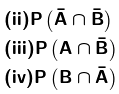Given: A and B are twoevents.

P (A) = 0.54, P (B) =0.69 and P (A ∩ B) = 0.35

By definition of P (Aor B) under axiomatic approach we know that:

P (A  B) = P (A) + P (B) – P (A ∩ B)

Now we have to find:

(i) P (A  B) = P (A) + P (B) – P (A ∩ B)

= 0.54 + 0.69 – 0.35

= 0.88

(ii) P(A′ ∩ B′) = P (A  B)′ {using De Morgan’s Law}

P (A′ ∩ B′)= 1 – P (A  B)

= 1 – 0.88

= 0.12

(iii) P(A ∩ B′) [This indicates only the part which is common with A and notB.

Hence this indicatesonly A]

P (only A) = P (A) – P(A ∩ B)

P(A ∩ B′) = P (A) – P (A ∩ B)

= 0.54 – 0.35

= 0.19

(iv) P(A′ ∩ B) [This indicates only the part which is common with B and notA.

Hence this indicatesonly B]

P (only B) = P (B) – P(A ∩ B)

P(A′ ∩ B) = P (B) – P (A ∩ B)

= 0.69 – 0.35

= 0.34

Question 3 : Fill in the blanks in the following table: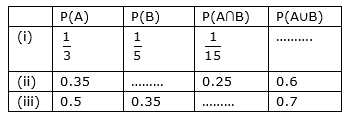(i) By definition ofP (A or B) under axiomatic approach we know that:

P (A  B) = P (A) + P (B) – P (A ∩ B)

By using data fromtable, we get:

P (A  B) = 1/3 + 1/5 – 1/15

= 8/15 – 1/15

= 7/15

(ii) By definition ofP (A or B) under axiomatic approach we know that:

P (A  B) = P (A) + P (B) – P (A ∩ B)

P (B) = P (A  B) + P (A ∩ B) – P (A)

By using data fromtable, we get:

P (B) = 0.6 +0.25 – 0.35

= 0.5

(iii) By definition ofP (A or B) under axiomatic approach we know that:

P (A  B) = P (A) + P (B) – P (A ∩ B)

P(A ∩ B) = P (B) + P (A) – P (A  B)

By using data fromtable, we get:

P(A ∩ B) = 0.5 + 0.35 – 0.7

= 0.15

Hence the table is: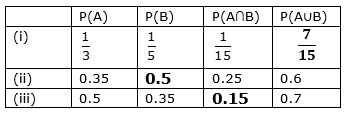Question 4 : If A and B are two events associated with a random experiment such that P (A) = 0.3, P (B) = 0.4 and P (A ∪ B) = 0.5, find P (A ∩ B).

Given: A and B are twoevents.

P (A) = 0.3, P (B) =0.5 and P (A  B) = 0.5

Now we need to find P(A ∩ B).

By definition of P (Aor B) under axiomatic approach (also called addition theorem) we know that:

P (A  B) = P (A) + P (B) – P (A ∩ B)

So, P(A ∩ B) = P (A) + P (B) – P (A  B)

P (A ∩ B) =0.3 + 0.4 – 0.5

= 0.7 – 0.5

= 0.2

P(A ∩ B) is 0.2

Question 5 : If A and B are two events associated with a random experiment such that P (A) = 0.5, P (B) = 0.3 and P (A ∩ B) = 0.2, find P (A ∪ B).

Given: A and B are twoevents.

P (A) = 0.5, P (B) =0.3 and P (A ∩ B) = 0.2

Now we need to find P(A  B).

By definition of P (Aor B) under axiomatic approach (also called addition theorem) we know that:

P (A  B) = P (A) + P (B) – P (A ∩ B)

So, P (A  B) = P (A) + P (B) – P (A ∩ B)

P (A  B) = 0.5 + 0.3 – 0.2

= 0.8 – 0.2

= 0.6

P (A  B) is 0.6

Question 6 :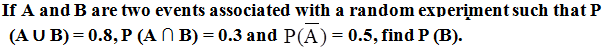Given: A and B are twoevents.

P (A′) = 0.5, P(A ∩ B) = 0.3 and P (A  B) = 0.8

Since, P (A′) = 1 – P(A)

P (A) = 1 – 0.5

= 0.5

Now we need to find P(B).

By definition of P (Aor B) under axiomatic approach (also called addition theorem) we know that:

P (A  B) = P (A) + P (B) – P (A ∩ B)

So, P (B) = P(A  B) + P(A ∩ B) – P (A)

P (B) = 0.8 + 0.3 –0.5

= 1.1 – 0.5

= 0.6

P (B) is 0.6

Question 7 : Given two mutually exclusive events A and B such that P (A) = 1/2 and P (B) = 1/3, find P (A or B).

Given: A and B are twomutually exclusive events.

P (A) = 1/2 and P (B)= 1/3

Now we need to find P(A ‘or’ B).

P (A or B) = P (A  B)

So by definition ofmutually exclusive events we know that:

P (A  B) = P (A) + P (B)

= 1/2 + 1/3

= 5/6

P (A  B) is 5/6

Question 8 : There are three events A, B, C one of which must and only one can happen, the odds are 8 to 3 against A, 5 to 2 against B, fins the odds against C.

As, out of 3 events A,B and C only one can happen at a time which means no event have anythingcommon.

We can say thatA, B and C are mutually exclusive events.

So, by definition ofmutually exclusive events we know that:

P (A  B  C) = P (A) + P (B) + P (C)

According to questionone event must happen.

So, A or B or C is asure event.

P (A  B  C) = 1 … Equation (1)

We need to find oddagainst C:

Given:

Odd against A = 8/3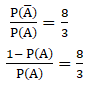8 P (A) = 3 – 3 P (A)

11 P (A) = 3

P (A) =3/11 … Equation (2)

Similarly, we aregiven with: Odd against B = 5/2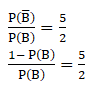5 P (B) = 2 – 2 P(B)

7 P (B) = 2

P (B)=2/7 …Equation (3)

From equation 1, 2 and3 we get:

P (C) = 1 – 3/11 – 2/7

= [77-21-22]/77

= 34/77

So, P (C′) = 1 –(34/77)

= 43/77

Odd against C: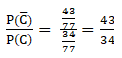Question 9 : One of the two events must happen. Given that the chance of one is two-third of the other, find the odds in favour of the other.

Let A and B are twoevents.

As, out of 2 events Aand B only one can happen at a time which means no event have anything common.

We can say thatA and B are mutually exclusive events.

So, by definition ofmutually exclusive events we know that:

P (A  B) = P (A) + P (B)

According to questionone event must happen.

A or B is a sureevent.

So, P (A  B) = P (A) + P (B) = 1 … Equation (1)

Given: P (A) = (2/3) P(B)

We have to find theodds in favour of B.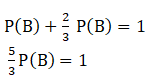P (B) = 3/5

So, P (B′) = 1 – 3/5

= 2/5

Odd in favour ofB: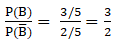Question 10 : A card is drawn at random from a well-shuffled deck of 52 cards. Find the probability of its being a spade or a king.

Given: As a card isdrawn from a deck of 52 cards.

Let ‘S’ denotes theevent of card being a spade and ‘K’ denote the event of card being King.

As we know that a deckof 52 cards contains 4 suits (Heart, Diamond, Spade and Club) each having 13cards. The deck has 4 king cards one from each suit.

We know thatprobability of an event E is given as-

By using the formula,

P (E) = favourableoutcomes / total possible outcomes

= n (E) / n (S)

Where, n (E) = numbersof elements in event set E

And n (S) = numbers ofelements in sample space.

Hence,

P (S) = n (spade) /total number of cards

= 13 / 52

= ¼

P (K) = 4/52

= 1/13

And P (S K) = 1/52

We need to find theprobability of card being spade or king, i.e.

P (Spade ‘or’ King) =P(S  K)

So, by definition of P(A or B) under axiomatic approach (also called addition theorem) we know that:

P (A  B) = P (A) + P (B) – P (A ∩ B)

So, P (S  K) = P (S) + P (K) – P (S ∩ K)

= ¼ + 1/13 – 1/52

= 17/52 – 1/52

= 16/52

= 4/13

P (S  K) = 4/13

Todays Deals### RD Chapter 33- Probability Ex-33.4 Contributorskrishan

Name:
Email:

# Latest News# 9000 interview questions in different categories